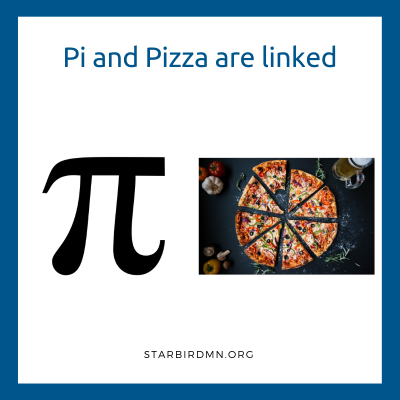Did you know…

The mathematical volume of pizza….is pizza.

If z = radius of a pizza, and

If a = height of a pizza, then

π * radius² * height = Pi * z * z * a = Pizza